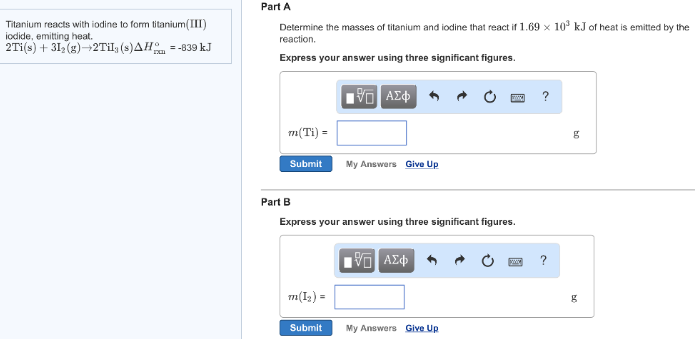# Problem: Titanium reacts with iodine to form titanium(III) iodide, emitting heat. 2Ti (s) + 3I2 (g) → 2TiI3 (s)           ΔH°rxn = 839 kJ Part ADetermine the masses of titanium and iodine that react if 1.69 x 103 kJ of heat is emitted by the reaction. Express your answer using three significant figures. Part BExpress your answer using three significant figures.

###### FREE Expert Solution
95% (56 ratings)###### Problem Details

Titanium reacts with iodine to form titanium(III) iodide, emitting heat.

2Ti (s) + 3I2 (g) → 2TiI3 (s)           ΔH°rxn = 839 kJ

Part A

Determine the masses of titanium and iodine that react if 1.69 x 103 kJ of heat is emitted by the reaction.

Part B